The purpose of the phase splitter stage is to produce two identical phase inverted output signals from the input signal. The two phase inverted signals are then passed to the push-pull power stage.

The most common schemas are the paraphase phase splitter, the long-tailed pair phase splitter, and the concertina phase splitter. For simplicity, here we will discuss the concertina phase splitter only. Despite its simplicity, a well-designed concertina phase splitter stage offers very high quality. Its only drawbacks might be that, as we will see later, it does not produce any gain on the input signal.

### 4.2.1    Concertina phase splitter

The basic schema of a concertina, or cathodyne, phase splitter is given in Figure 25. Two resistors of exactly the same resistance Ra=Rk are connected, respectively, from the high-tension V+ to the anode, and from the cathode to the ground. In this way, the two resistors, along with the vacuum tube internal resistance, form a voltage divider. When grid voltage increases, the resistance offered by the vacuum tube decreases. When the vacuum tube resistance decreases, the voltage at the anode decrease and the voltage at the cathode increases of exactly the same amount, provided the two resistors are perfectly matched. Similarly, when the grid voltage decreases, the anode and the cathode voltages, respectively increase and decrease of exactly the same amount. Consequently, two phase-inverted signals of exactly the same amplitude are taken at the anode and the cathode of the vacuum tube. These two signals are passed to the push-pull vacuum tubes of the next stage.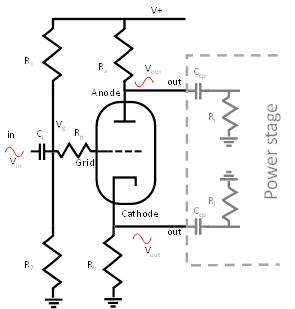Figure 25: Basic schema of a Concertina phase splitter.The concertina phase splitter uses two resistors of the same resistance Rk=Ra connected to the anode and to the cathode of a vacuum tube. Voltage variations of at the anode and at the cathode of the vacuum tube, due to a signal applied to the grid, are of the same amplitude and have inverted phases. A voltage divider, built with appropriate values of R1 and R2, is used to provide the gird with the correct bias voltage. The coupling capacitor Cc is used to isolate the previous stage from the bias voltage applied to the grid.

The grid bias voltage should be, as usual, negative with respect to the cathode voltage. In this configuration, the cathode voltage is higher than ground due to the effect of the cathode resistor Rk. Therefore, the voltage Vg should be lower, than the cathode voltage, of an amount equal to the wanted bias voltage. Bias voltage to the vacuum tube grid can be obtained using a voltage divider composed of the resistors R1 and R2.

 Example 9: Biasing the Concertina phase splitter Let Ib be the wanted bias current. The quiescent cathode voltage is Vk=Rk×Ib. Suppose we want a bias current Ib of 1 mA, corresponding to the red spot in Figure 26, and that the cathode resistance Rk is 100K Ohm. The cathode voltage is Vk=100K Ohm × 1 mA= 100V. Given that Ra is equal to Rk, the voltage drop, produced by the anode resistor, is also 100V and the anode voltage is Va=380V-100V=280V. Consequently, the anode to cathode voltage is Va– Vk=280V-100V=180V. According to Figure 26, the bias voltage applied to the grid should be approximatively 1.4V below the cathode voltage. This corresponds to 100V-1.4V=98.6V from ground. To compute the correct values for R1 and R2 we can use the voltage divider equation: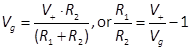. Suppose high-tension V+ is 380V, the ratio between the two resistors must be (380V/98,6V)-1≈ 2,85. Here large values have to be used, to avoid dissipating too much power. For example, R1=1,2M Ohm and R2=560K Ohm are values that give a ratio very close to what we need.

The grid stopper resistor Rg has the purpose of blocking very high frequencies and parasitic oscillations, by creating a low-pass filter together with the internal vacuum tube capacitance, due to the Miller effect, as already discussed in Section 4.1.1. The grid stopper resistor must be soldered directly on the pin of the vacuum tube socket. For instance, values around 47K Ohm are generally used for vacuum tubes like the 12AX7.

The coupling capacitor Cc isolates previous stage from the bias voltage applied to the grid. The coupling capacitor together with R1 and R2, seen as parallel resistors when handling an AC signal, form a high-pass filter. Cc has to be chosen according to the wanted low cut-off frequency.

 Example 10: Inter-stage coupling capacitor in a concertina phase splitter Suppose R1=1,2M Ohm, R2=560K Ohm, and we want a cut-off frequency at 7 Hz, given that R1 and R2 in parallel are R=382K Ohm, we have: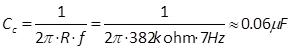### 4.2.2    DC and AC loadline of a concertina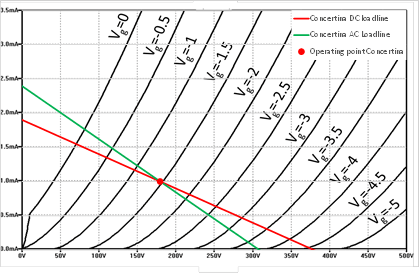Figure 26: DC and AC loadline of a concertina phase splitter.The concertina phase splitter has two loadlines. The DC loadline has to be considered when the vacuum tube is in a quiescent state. In this case, just the anode and cathode resistors contribute to the overall load seen by the vacuum tube. When a signal is applied to the grid, the signals at the anode and cathode of the vacuum tube go through the coupling capacitors Ccp and from these to the grid leak resistors of the power stage, which are now parallel to the anode and cathode resistors. The total load seen by the vacuum tube is reduced and the AC loadline has to be considered in this case.
 Example 11: DC and AC loadlines in a concertina phase splitter Suppose that Ra=Rk=100K Ohm, that the two grid leaks of the power stage are Rl=180K Ohm, and that the high-tension is V+=380V. The total DC load is 200K Ohm and produces the DC loadline depicted by the red line in Figure 26. The AC load seen, respectively at the anode and at the cathode, is the result of 100K Ohm in parallel with 180K Ohm, which is approximately 64K Ohm. The total AC load in this case is 128K Ohm, which gives the green AC loadline in Figure 26.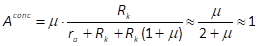.